## Things Are Getting Complex

### Things Are Getting Complex

As if things weren't complicated enough, it's time to learn about other types of numbers. Yes, there are other types of numbers. The numbers we've been adding, subtracting, multiplying, and dividing for years have been in the group called real numbers. Real numbers are just the group of all rational and irrational numbers with whom we've already been introduced.

Believe it or not, in a land not so far, far away, there exists another group known as imaginary numbers. These numbers include a magical number i. Yes, that's correct. We said the number i, which is defined as the square root of -1.

So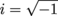, and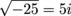, and so on. While we won't go absolutely crazy with these imaginary numbers in this chapter, they're an important part of your newfound radical knowledge. At least you get the t-shirt joke now.

To round out this mind-blowing Shmexperience, we have one more type of number for you to consider. This is the group of complex numbers. Complex numbers can be, well, complex. Thankfully, you have us to explain.

Complex numbers are really just a combination of a real number and an imaginary number added together. These typically take this form:

a + bi

...where a and b are both real numbers. The a is known as the real part, while the bi is the imaginary part.

While we could very easily fill section after section all about complex numbers, for now we'll mostly focus on simplifying them. This will make our mathematical lives simpler later down the road. You're welcome.

### Sample Problem

Simplify the following: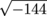.

This is a pretty easy sample problem to start things out. Since the square root of 144 is just 12 and the square root of -1 is i, we get: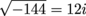### Sample Problem

Simplify the following: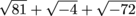.

Now this is more like it. Let's go term by term. First, we'll take care of √81.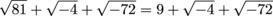Next, we can simplify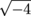. This is a lot like the previous sample problem: the square root of 4 is 2, and the square root of -1 is i.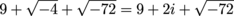Looks like our final answer is going to be a complex number. However, we need to simplify our third term. This means we'll need to write it in simple radical form.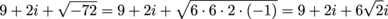Almost finished. Typically when we have multiple imaginary terms, we'll want to write them together. In this case, we can factor out i from the 2 and 6√2, so we end up with…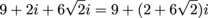### Sample Problem

Simplify the following: i 7.

This is a little change of pace, but certainly nothing we can't handle. First, let's analyze what we know. If i = √-1, then it makes perfect sense that i2 = (√-1)2 or i2 = -1. This is great news, because we can break i 7 down into a bunch of little i2's.

i 7 = i2i2i2i

Then, if we just substitute in a -1 for each i2:

i2i2i2i = (-1)(-1)(-1)i

Finally we can simplify and things end up looking pretty calm, cool, and collected.

(-1)(-1)(-1)i = -i

### Sample Problem

Simplify the following: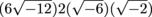.

We could take one of two approaches to solve this one. First, we could break down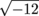into its simple radical form. However, we think that multiplying the whole numbers with each other and the radicals with each other is gonna save us some heartache and pain down the stretch.

Multiply that 6 and 2 together, then multiply all the radical stuff together: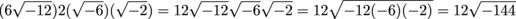Bonus! We don't even need to use simple radical form at all now. We can just go ahead and simplify our square root and get our final answer.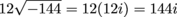Love it. One more.

### Sample Problem

Simplify the following: 5i 3√(-45).

It's time for more multiplication, but now there's a pesky little i involved. #mathstudentprobs

Let's go to work with that square root first.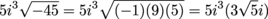Now that we've turned our square root into simple radical form, this problem becomes nothing more than a little multiplication.Of course, since i2 is still -1 just like it was in the previous problem, things work out pretty well. And that, ladies and gents, deserves an old-fashioned court storming. After all, what's more fun than storming the court after a big upset victory?